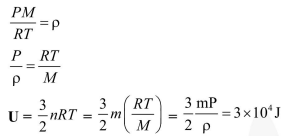# One kg of a diatomic gas`
Question:

One kg of a diatomic gas is at a pressure of $8 \times 10^{4} \mathrm{~N} / \mathrm{m}^{2}$. The density of the gas is $4 \mathrm{~kg} / \mathrm{m}^{3}$. What is the energy of the gas due to its thermal motion ?

1. $6 \times 10^{4} \mathrm{~J}$

2. $7 \times 10^{4} \mathrm{~J}$

3. $3 \times 10^{4} \mathrm{~J}$

4. $5 \times 10^{4} \mathrm{~J}$

Correct Option: , 3

Solution: